# Vision Transformer影象分類(MindSpore實現)

## Vision Transformer（ViT）簡介

ViT則是自然語言處理和計算機視覺兩個領域的融合結晶。在不依賴卷積操作的情況下，依然可以在影象分類任務上達到很好的效果。

### 模型結構

ViT模型的主體結構是基於Transformer模型的Encoder部分（部分結構順序有調整，如：normalization的位置與標準Transformer不同），其結構圖如下：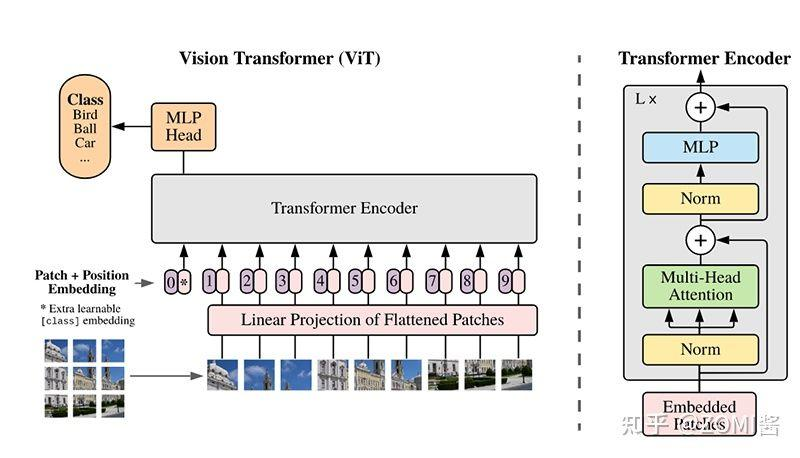### 模型特點

ViT模型是應用於影象分類領域。因此，其模型結構相較於傳統的Transformer有以下幾個特點：

1. 資料集的原影象被劃分為多個patch後，將二維patch（不考慮channel）轉換為一維向量，再加上類別向量與位置向量作為模型輸入。

## 環境準備與資料讀取

```.ImageNet/ ├── ILSVRC2012_devkit_t12.tar.gz ├── train/ └── val/```

\

``` from mindspore import context from mindvision.classification.dataset import ImageNet

context.set_context(mode=context.GRAPH_MODE, device_target='GPU')

data_url = './ImageNet/' resize = 224 batch_size = 16

dataset_train = ImageNet(data_url, split="train", shuffle=True, resize=resize, batch_size=batch_size, repeat_num=1, num_parallel_workers=1).run() ```

## 模型解析

### Transformer基本原理

Transformer模型源於2017年的一篇文章。在這篇文章中提出的基於Attention機制的編碼器-解碼器型結構在自然語言處理領域獲得了巨大的成功。模型結構如下圖所示：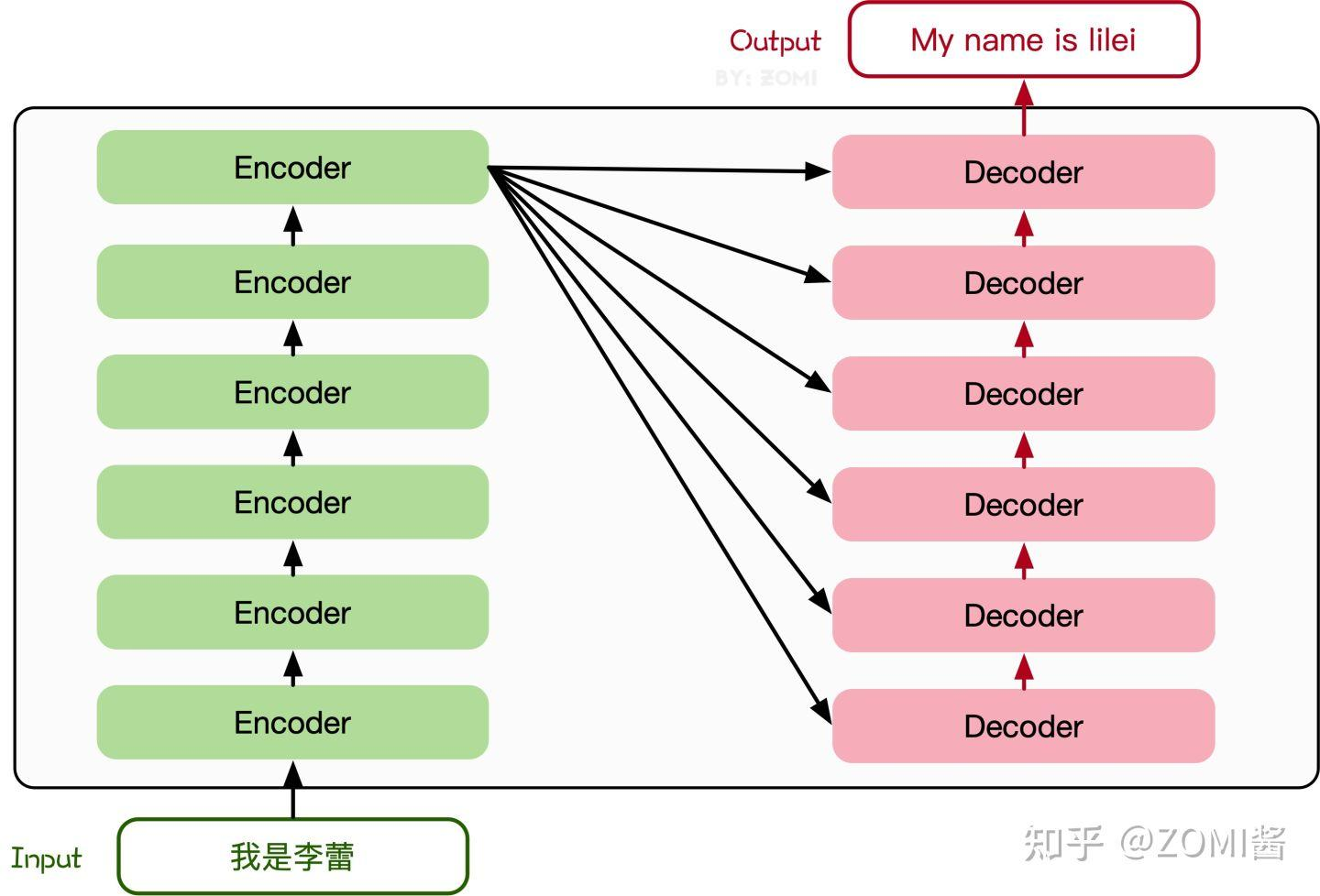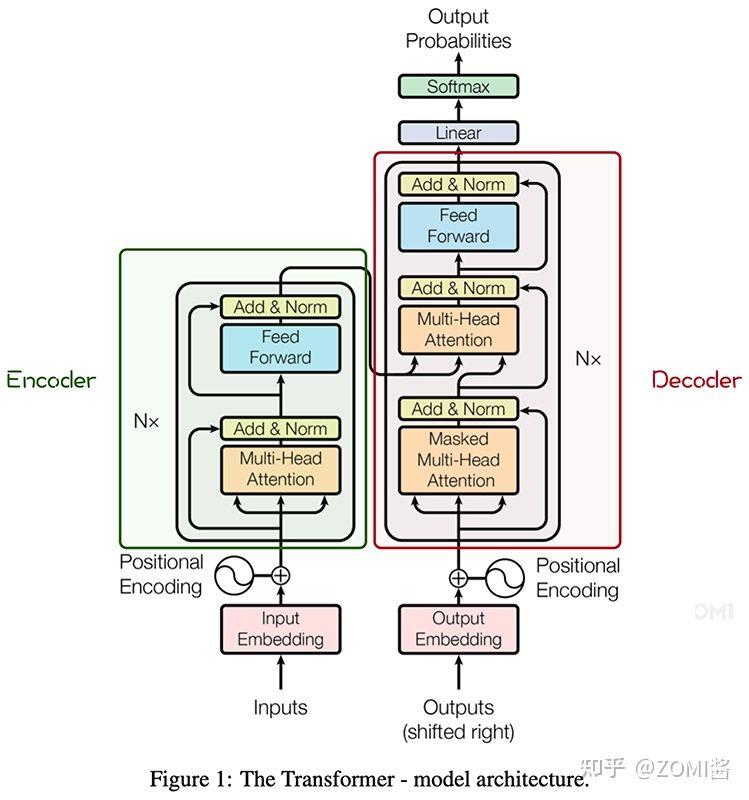### Attention模組

1. 最初的輸入向量首先會經過Embedding層對映成Q（Query），K（Key），V（Value）三個向量，由於是並行操作，所以程式碼中是對映成為dim x 3的向量然後進行分割，換言之，如果你的輸入向量為一個向量序列（ 1x1 1x1， 2x2 2x2， 3x3 3x3），其中的 1x1 1x1， 2x2 2x2， 3x3 3x3都是一維向量，那麼每一個一維向量都會經過Embedding層映射出Q，K，V三個向量，只是Embedding矩陣不同，矩陣引數也是通過學習得到的。這裡大家可以認為，Q，K，V三個矩陣是發現向量之間關聯資訊的一種手段，需要經過學習得到，至於為什麼是Q，K，V三個，主要是因為需要兩個向量點乘以獲得權重，又需要另一個向量來承載權重向加的結果，所以，最少需要3個矩陣，也是論文作者經過實驗得出的結論。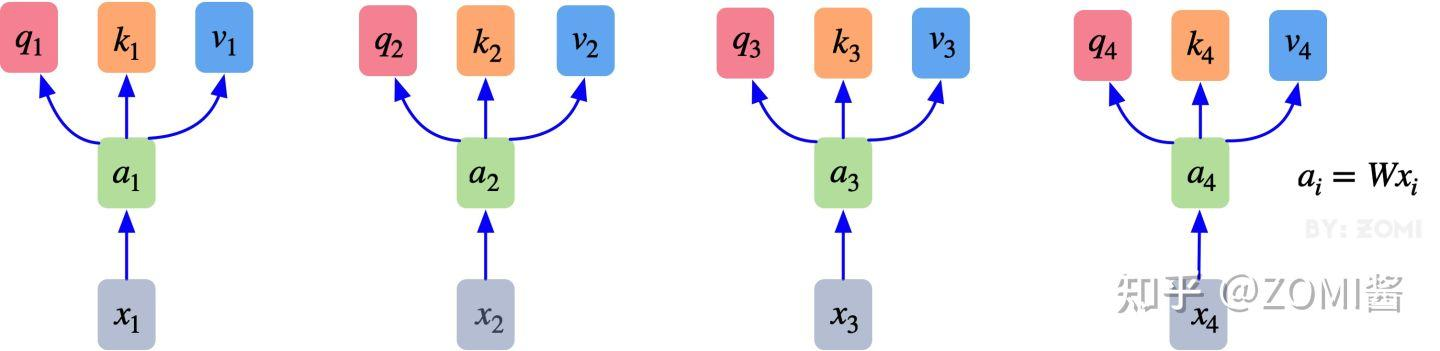1. 自注意力機制的自注意主要體現在它的Q，K，V都來源於其自身，也就是該過程是在提取輸入的不同順序的向量的聯絡與特徵，最終通過不同順序向量之間的聯絡緊密性（Q與K乘積經過softmax的結果）來表現出來。Q，K，V得到後就需要獲取向量間權重，需要對Q和K進行點乘併除以維度的平方根 ⎯⎯√d ⎯⎯√d，對所有向量的結果進行Softmax處理，通過公式(2)的操作，我們獲得了向量之間的關係權重。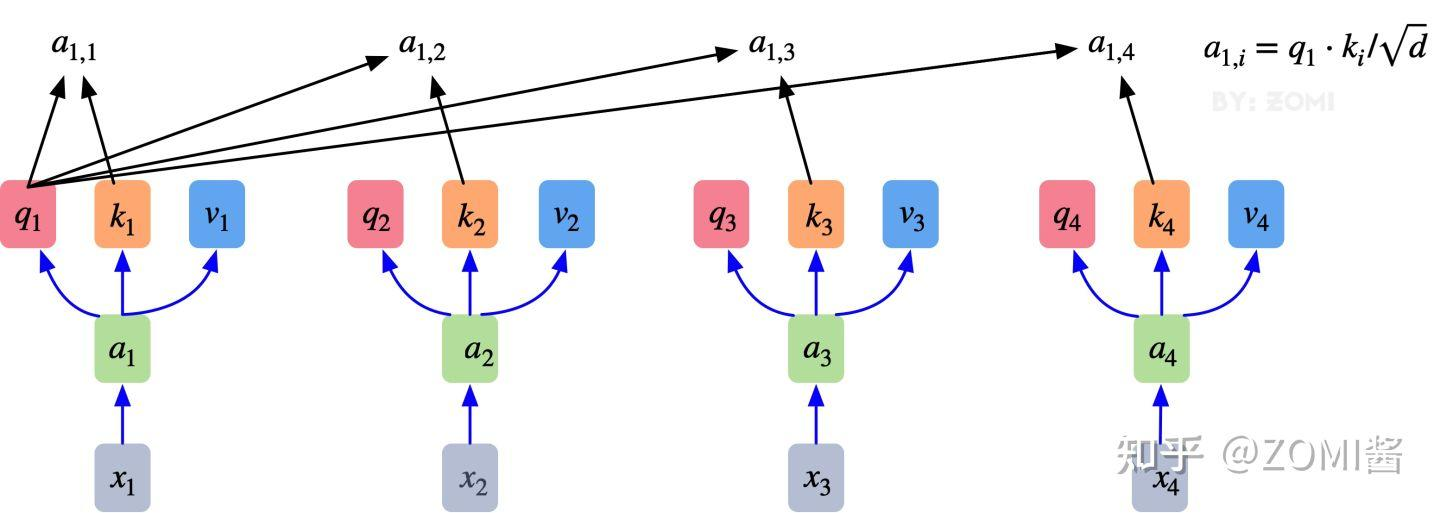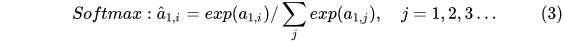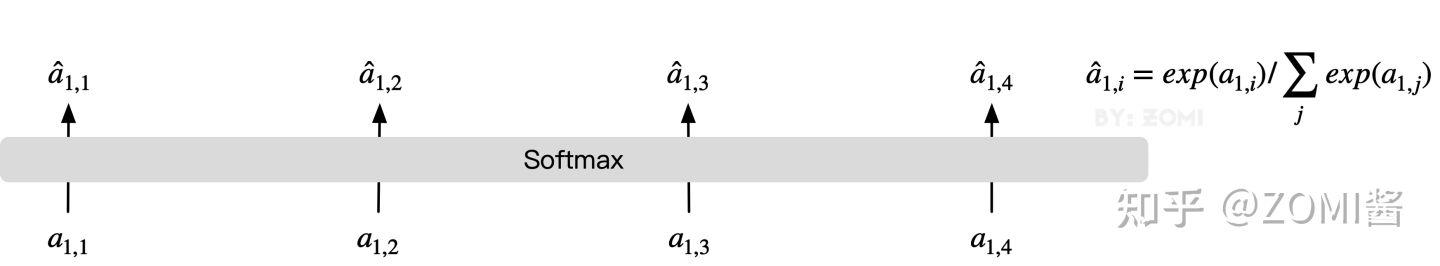3.其最終輸出則是通過V這個對映後的向量與QK經過Softmax結果進行weight sum獲得，這個過程可以理解為在全域性上進行自注意表示。每一組QKV最後都有一個V輸出，這是Self-Attention得到的最終結果，是當前向量在結合了它與其他向量關聯權重後得到的結果。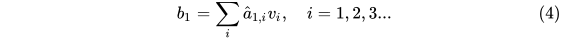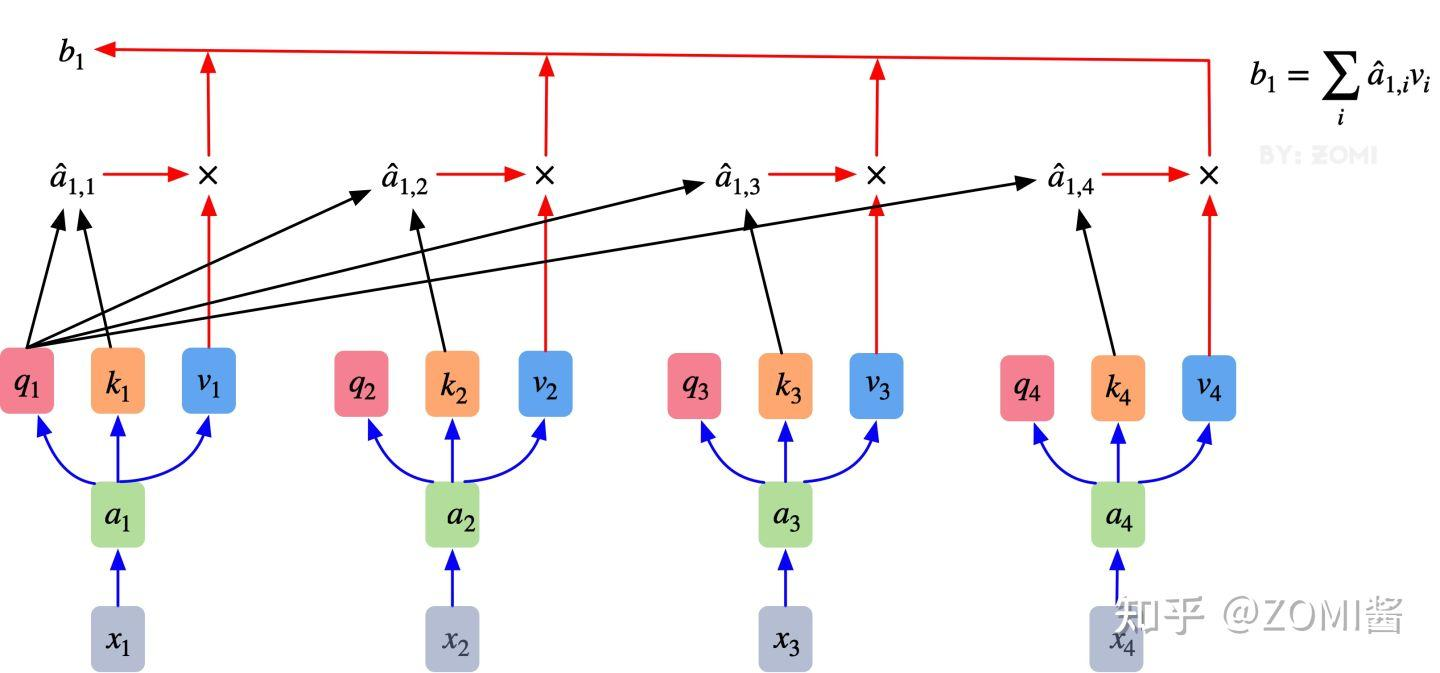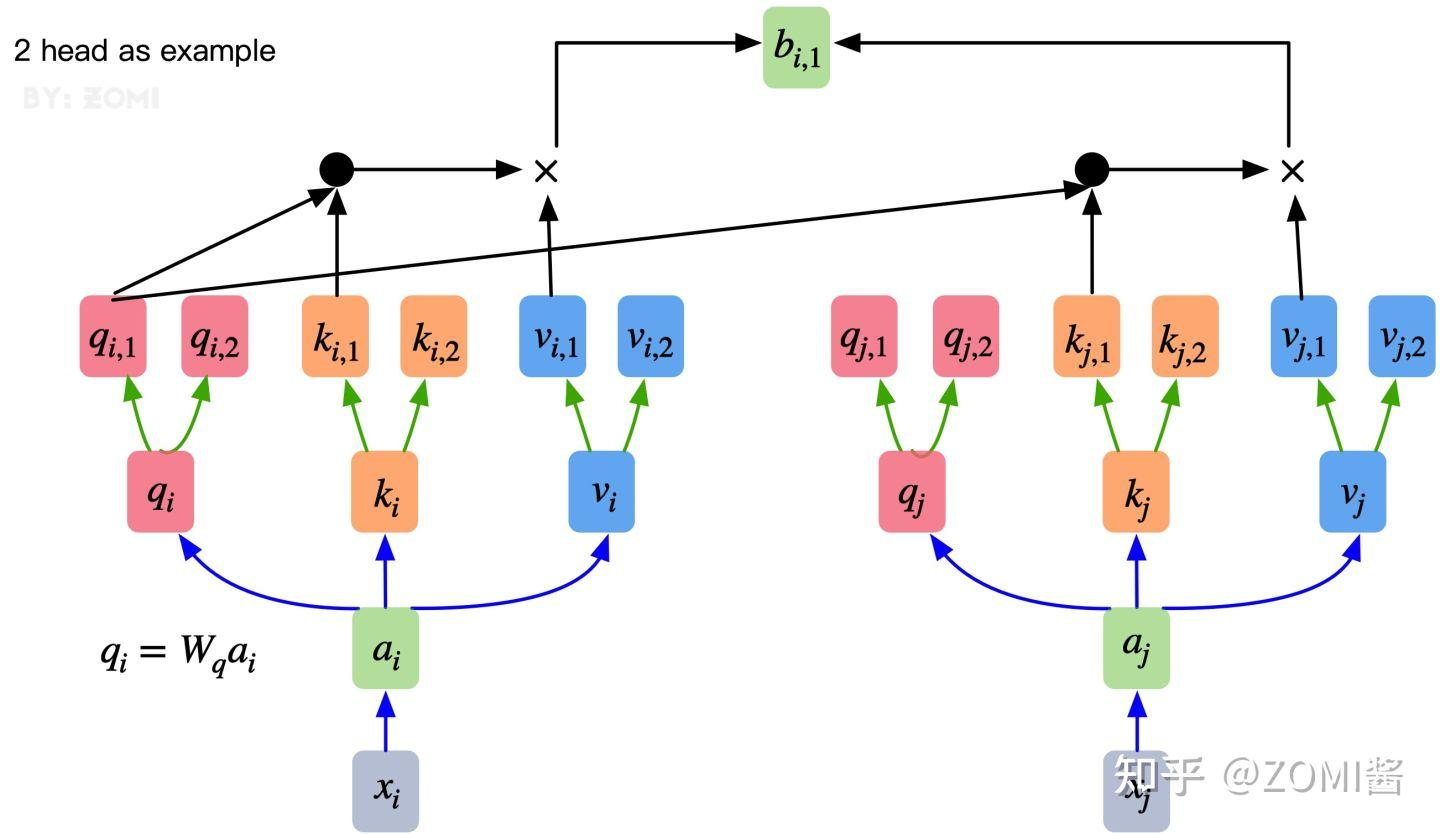``` import mindspore.nn as nn

class Attention(nn.Cell): def init(self, dim: int, num_heads: int = 8, keep_prob: float = 1.0, attention_keep_prob: float = 1.0): super(Attention, self).init()

``````    self.num_heads = num_heads

self.qkv = nn.Dense(dim, dim * 3)
self.attn_drop = nn.Dropout(attention_keep_prob)
self.out = nn.Dense(dim, dim)
self.out_drop = nn.Dropout(keep_prob)

self.mul = P.Mul()
self.reshape = P.Reshape()
self.transpose = P.Transpose()
self.unstack = P.Unstack(axis=0)
self.attn_matmul_v = P.BatchMatMul()
self.q_matmul_k = P.BatchMatMul(transpose_b=True)
self.softmax = nn.Softmax(axis=-1)

def construct(self, x):
"""Attention construct."""
b, n, c = x.shape

# 最初的輸入向量首先會經過Embedding層對映成Q(Query)，K(Key)，V(Value)三個向量
# 由於是並行操作，所以程式碼中是對映成為dim*3的向量然後進行分割
qkv = self.qkv(x)

qkv = self.transpose(qkv, (2, 0, 3, 1, 4))
q, k, v = self.unstack(qkv)

# 自注意力機制的自注意主要體現在它的Q，K，V都來源於其自身
# 也就是該過程是在提取輸入的不同順序的向量的聯絡與特徵
# 最終通過不同順序向量之間的聯絡緊密性（Q與K乘積經過softmax的結果）來表現出來
attn = self.q_matmul_k(q, k)
attn = self.mul(attn, self.scale)
attn = self.softmax(attn)
attn = self.attn_drop(attn)

# 其最終輸出則是通過V這個對映後的向量與QK經過Softmax結果進行weight sum獲得
# 這個過程可以理解為在全域性上進行自注意表示
out = self.attn_matmul_v(attn, v)
out = self.transpose(out, (0, 2, 1, 3))
out = self.reshape(out, (b, n, c))
out = self.out(out)
out = self.out_drop(out)

return out
``````

```

### Transformer Encoder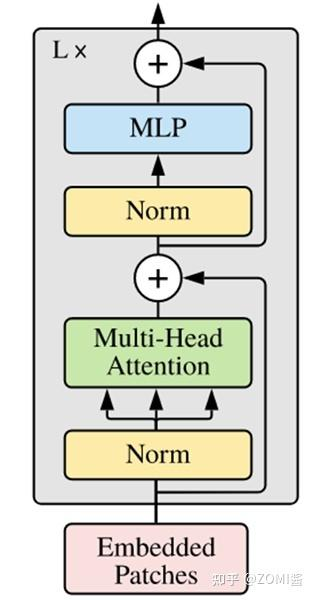1. ViT模型中的基礎結構與標準Transformer有所不同，主要在於Normalization的位置是放在Self-Attention和Feed Forward之前,其他結構如Residual Connection，Feed Forward，Normalization都如Transformer中所設計。
2. 從transformer結構的圖片可以發現，多個子encoder的堆疊就完成了模型編碼器的構建，在ViT模型中，依然沿用這個思路，通過配置超引數num_layers，就可以確定堆疊層數。
3. Residual Connection，Normalization的結構可以保證模型有很強的擴充套件性（保證資訊經過深層處理不會出現退化的現象，這是Residual Connection的作用），Normalization和dropout的應用可以增強模型泛化能力。

``` class TransformerEncoder(nn.Cell): def init(self, dim: int, num_layers: int, num_heads: int, mlp_dim: int, keep_prob: float = 1., attention_keep_prob: float = 1.0, drop_path_keep_prob: float = 1.0, activation: nn.Cell = nn.GELU, norm: nn.Cell = nn.LayerNorm): super(TransformerEncoder, self).init() layers = []

``````    # 從vit_architecture圖可以發現，多個子encoder的堆疊就完成了模型編碼器的構建
# 在ViT模型中，依然沿用這個思路，通過配置超引數num_layers，就可以確定堆疊層數
for _ in range(num_layers):
normalization1 = norm((dim,))
normalization2 = norm((dim,))
attention = Attention(dim=dim,
keep_prob=keep_prob,
attention_keep_prob=attention_keep_prob)

feedforward = FeedForward(in_features=dim,
hidden_features=mlp_dim,
activation=activation,
keep_prob=keep_prob)

# ViT模型中的基礎結構與標準Transformer有所不同
# 主要在於Normalization的位置是放在Self-Attention和Feed Forward之前
# 其他結構如Residual Connection，Feed Forward，Normalization都如Transformer中所設計
layers.append(
nn.SequentialCell([
# Residual Connection，Normalization的結構可以保證模型有很強的擴充套件性
# 保證資訊經過深層處理不會出現退化的現象，這是Residual Connection的作用
# Normalization和dropout的應用可以增強模型泛化能力
ResidualCell(nn.SequentialCell([normalization1,
attention])),

ResidualCell(nn.SequentialCell([normalization2,
feedforward]))
])
)
self.layers = nn.SequentialCell(layers)

def construct(self, x):
"""Transformer construct."""
return self.layers(x)
``````

```

### ViT模型的輸入

1. 通過將輸入影象在每個channel上劃分為1616個patch，這一步是通過卷積操作來完成的，當然也可以人工進行劃分，但卷積操作也可以達到目的同時還可以進行一次而外的資料處理； 例如一幅輸入224 x 224的影象，首先經過卷積處理得到16 x 16個patch，那麼每一個patch的大小就是14 x 14。*
2. 再將每一個patch的矩陣拉伸成為一個1維向量，從而獲得了近似詞向量堆疊的效果。上一步得道的14 x 14的patch就轉換為長度為196的向量。

``` class PatchEmbedding(nn.Cell): MIN_NUM_PATCHES = 4 def init(self, image_size: int = 224, patch_size: int = 16, embed_dim: int = 768, input_channels: int = 3): super(PatchEmbedding, self).init()

``````    self.image_size = image_size
self.patch_size = patch_size
self.num_patches = (image_size // patch_size) ** 2

# 通過將輸入影象在每個channel上劃分為16*16個patch
self.conv = nn.Conv2d(input_channels, embed_dim, kernel_size=patch_size, stride=patch_size, has_bias=True)
self.reshape = P.Reshape()
self.transpose = P.Transpose()

def construct(self, x):
"""Path Embedding construct."""
x = self.conv(x)
b, c, h, w = x.shape

# 再將每一個patch的矩陣拉伸成為一個1維向量，從而獲得了近似詞向量堆疊的效果；
x = self.reshape(x, (b, c, h * w))
x = self.transpose(x, (0, 2, 1))

return x
``````

```

1. class_embedding主要借鑑了BERT模型的用於文字分類時的思想，在每一個word vector之前增加一個類別值，通常是加在向量的第一位,上一步得到的196維的向量加上class_embedding後變為197維。
2. 增加的class_embedding是一個可以學習的引數，經過網路的不斷訓練，最終以輸出向量的第一個維度的輸出來決定最後的輸出類別；由於輸入是16 x 16個patch，所以輸出進行分類時是取 16 x 16個class_embedding進行分類。
3. pos_embedding也是一組可以學習的引數，會被加入到經過處理的patch矩陣中。
4. 由於pos_embedding也是可以學習的引數，所以它的加入類似於全連結網路和卷積的bias。這一步就是創造一個長度維197的可訓練向量加入到經過class_embedding的向量中。

### 整體構建ViT

``` from typing import Optional

class ViT(nn.Cell): def init(self, image_size: int = 224, input_channels: int = 3, patch_size: int = 16, embed_dim: int = 768, num_layers: int = 12, num_heads: int = 12, mlp_dim: int = 3072, keep_prob: float = 1.0, attention_keep_prob: float = 1.0, drop_path_keep_prob: float = 1.0, activation: nn.Cell = nn.GELU, norm: Optional[nn.Cell] = nn.LayerNorm, pool: str = 'cls') -> None: super(ViT, self).init()

``````    self.patch_embedding = PatchEmbedding(image_size=image_size,
patch_size=patch_size,
embed_dim=embed_dim,
input_channels=input_channels)
num_patches = self.patch_embedding.num_patches

# 此處增加class_embedding和pos_embedding，如果不是進行分類任務
# 可以只增加pos_embedding，通過pool引數進行控制
self.cls_token = init(init_type=Normal(sigma=1.0),
shape=(1, 1, embed_dim),
dtype=ms.float32,
name='cls',

# pos_embedding也是一組可以學習的引數，會被加入到經過處理的patch矩陣中
self.pos_embedding = init(init_type=Normal(sigma=1.0),
shape=(1, num_patches + 1, embed_dim),
dtype=ms.float32,
name='pos_embedding',

# axis=1定義了會在向量的開頭加入class_embedding
self.concat = P.Concat(axis=1)

self.pool = pool
self.pos_dropout = nn.Dropout(keep_prob)
self.norm = norm((embed_dim,))
self.tile = P.Tile()
self.transformer = TransformerEncoder(dim=embed_dim,
num_layers=num_layers,
mlp_dim=mlp_dim,
keep_prob=keep_prob,
attention_keep_prob=attention_keep_prob,
drop_path_keep_prob=drop_path_keep_prob,
activation=activation,
norm=norm)

def construct(self, x):
"""ViT construct."""
x = self.patch_embedding(x)

# class_embedding主要借鑑了BERT模型的用於文字分類時的思想
# 在每一個word vector之前增加一個類別值，通常是加在向量的第一位
cls_tokens = self.tile(self.cls_token, (x.shape, 1, 1))
x = self.concat((cls_tokens, x))
x += self.pos_embedding

x = self.pos_dropout(x)
x = self.transformer(x)
x = self.norm(x)

# 增加的class_embedding是一個可以學習的引數，經過網路的不斷訓練
# 最終以輸出向量的第一個維度的輸出來決定最後的輸出類別；
x = x[:, 0]

return x
``````

```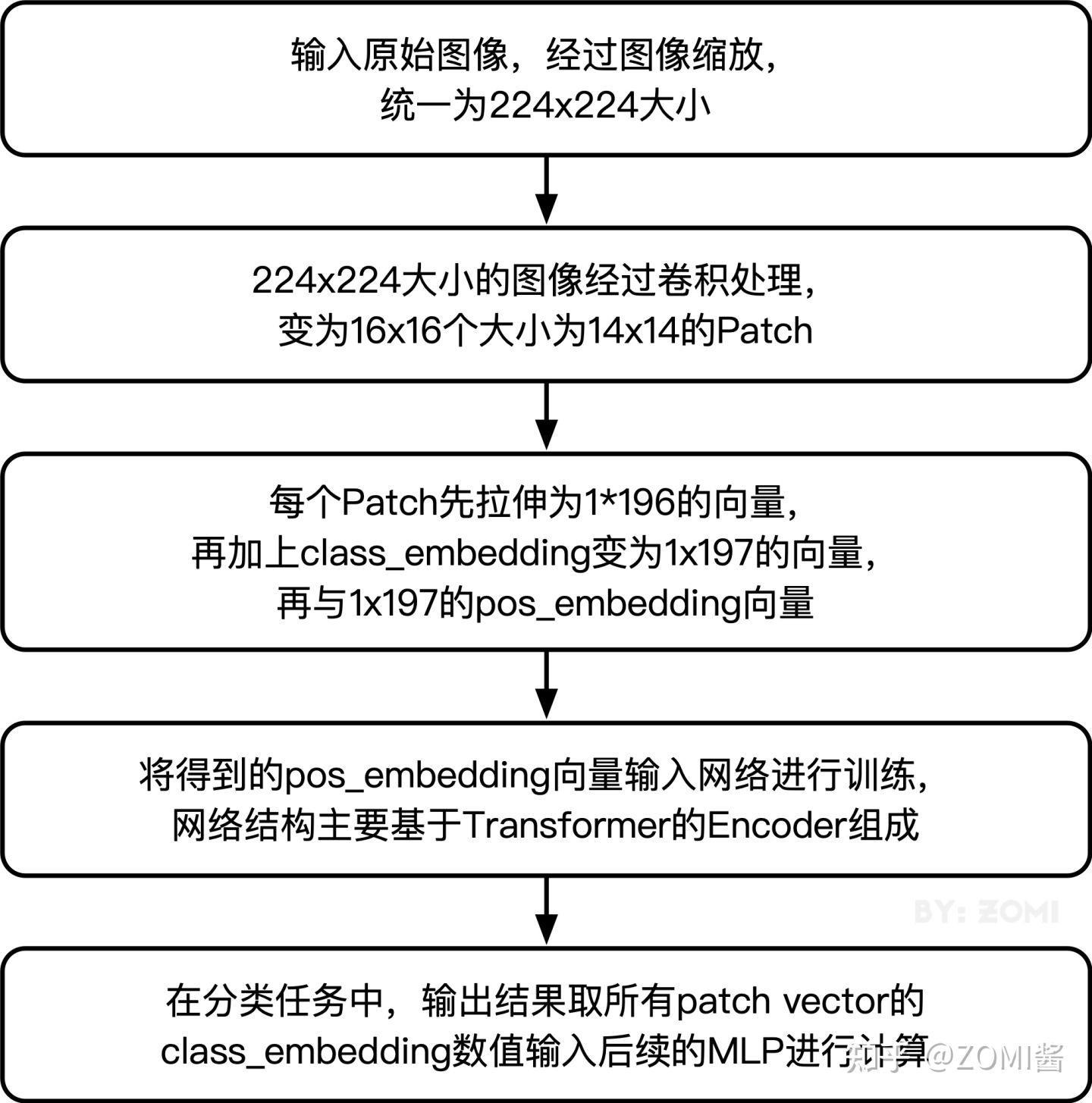``` import mindspore.nn as nn from mindspore.train import Model from mindspore.train.callback import ModelCheckpoint, CheckpointConfig

from mindvision.classification.models import vit_b_16 from mindvision.engine.callback import LossMonitor from mindvision.engine.loss import CrossEntropySmooth

# 定義超引數

epoch_size = 10 momentum = 0.9 step_size = dataset_train.get_dataset_size() num_classes = 1000

# 構建模型

network = vit_b_16(num_classes=num_classes, image_size=resize, pretrained=True)

# 定義遞減的學習率

lr = nn.cosine_decay_lr(min_lr=float(0), max_lr=0.003, total_step=epoch_size * step_size, step_per_epoch=step_size, decay_epoch=90)

# 定義損失函式

network_loss = CrossEntropySmooth(sparse=True, reduction="mean", smooth_factor=0.1, classes_num=num_classes)

# 設定checkpoint

ckpt_config = CheckpointConfig(save_checkpoint_steps=step_size, keep_checkpoint_max=100) ckpt_callback = ModelCheckpoint(prefix='vit_b_16', directory='./ViT', config=ckpt_config)

# 初始化模型

model = Model(network, loss_fn=network_loss, optimizer=network_opt, metrics={"acc"})

# 訓練

model.train(epoch_size, dataset_train, callbacks=[ckpt_callback, LossMonitor(lr)], dataset_sink_mode=False) ```

```Epoch:[ 0/ 10], step:[ 1/80072], loss:[1.963/1.963], time:8171.241 ms, lr:0.00300 Epoch:[ 0/ 10], step:[ 2/80072], loss:[7.809/4.886], time:769.321 ms, lr:0.00300 Epoch:[ 0/ 10], step:[ 3/80072], loss:[8.851/6.208], time:779.355 ms, lr:0.00300 .... Epoch:[ 9/ 10], step:[80070/80072], loss:[1.112/6.657], time:780.714 ms, lr:0.00240 Epoch:[ 9/ 10], step:[80071/80072], loss:[1.111/6.708], time:781.860 ms, lr:0.00240 Epoch:[ 9/ 10], step:[80072/80072], loss:[1.102/6.777], time:782.859 ms, lr:0.00240```

### 模型驗證

``` dataset_analyse = ImageNet(data_url, split="val", num_parallel_workers=1, resize=resize, batch_size=batch_size) dataset_eval = dataset_analyse.run()

network = vit_b_16(num_classes=num_classes, image_size=resize, pretrained=True)

network_loss = CrossEntropySmooth(sparse=True, reduction="mean", smooth_factor=0.1, classes_num=num_classes)

# 定義評價指標

eval_metrics = {'Top_1_Accuracy': nn.Top1CategoricalAccuracy(), 'Top_5_Accuracy': nn.Top5CategoricalAccuracy()}

model = Model(network, network_loss, metrics=eval_metrics)

# 評估模型

result = model.eval(dataset_eval) print(result) ```

`{'Top_1_Accuracy': 0.73524, 'Top_5_Accuracy': 0.91756}`

### 模型推理

``` import mindspore.dataset.vision.c_transforms as transforms

# 資料預處理操作

def infer_transform(dataset, columns_list, resize):

``````mean = [0.485 * 255, 0.456 * 255, 0.406 * 255]
std = [0.229 * 255, 0.224 * 255, 0.225 * 255]

trans = [transforms.Decode(),
transforms.Resize([resize, resize]),
transforms.Normalize(mean=mean, std=std),
transforms.HWC2CHW()]

dataset = dataset.map(operations=trans,
input_columns=columns_list,
num_parallel_workers=1)
dataset = dataset.batch(1)

return dataset
``````

```

``` import numpy as np

import mindspore.dataset as ds from mindspore import Tensor

# 讀取推理圖片

image_list, image_label = read_dataset('./infer') columns_list = ('image', 'label')

dataset_infer = ds.GeneratorDataset(DatasetGenerator(image_list, image_label), column_names=list(columns_list), num_parallel_workers=1)

dataset_infer = infer_transform(dataset_infer, columns_list, resize)

# 讀取資料進行推理

for i, image in enumerate(dataset_infer.create_dict_iterator(output_numpy=True)): image = image["image"] image = Tensor(image) prob = model.predict(image) label = np.argmax(prob.asnumpy(), axis=1)

``````predict = dataset_analyse.index2label[int(label)]
output = {int(label): predict}
print(output)
show_result(img=image_list[i], result=output, out_file=image_list[i])
``````

```

`{236: 'Doberman'}`## 引用

 Dosovitskiy, Alexey, et al. "An image is worth 16x16 words: Transformers for image recognition at scale." arXiv preprint arXiv:2010.11929 (2020).

 Vaswani, Ashish, et al. "Attention is all you need."Advances in Neural Information Processing Systems. (2017).

「其他文章」